# Research on the Condition Model of Drilling Fluid Non-retention in Eccentric Annulus

Research on the Condition Model of Drilling Fluid Non-retention in Eccentric Annulus

Feng Fuping Ai Chi  Xu Haisu  Cui Zhihua  Gao Changlong

Northeast Petroleum University, No.149 Fazhan Road, High-tech Development Zone, Daqing 163318, China

Page:
9-16
|
DOI:
https://doi.org/10.18280/ijht.330102
N/A
|
Accepted:
N/A
|
Published:
31 March 2015
| Citation

OPEN ACCESS

Abstract:

The drilling fluid that is retained in the annulus may become the channeling path for oil, gas and water in the formation. Therefore, ensuring drilling fluid non-retention during cementing is an effective method to improve the annular sealing quality. In order to solve the problem that precious researches can’t obtain the conditions of drilling fluid non-retention on the casing and well walls in eccentric annulus, this paper establishes a non-retention condition model through mechanism analysis of displacing fluid elements. Furthermore, several rules are obtained on the basis of the calculation results: for the retention range of drilling fluid, it becomes decreasing gradually on the casing and well walls as casing eccentricity increases, and the retention degree in the annular narrow gap will be more severe. Moreover, for the drilling fluid in the annular wide gap, the critical yield stress of drilling fluid non-retention and the difference of critical yield stress between casing and well walls are increasing. But the opposite results will obtain in the annular narrow gap. So, we can conclude that a better cementing effect can be obtained with the adjustment of displacing fluid properties and construction parameters on the basis of drilling fluid non-retention in the annular narrow gap.

Keywords:

eccentric annulus, non-retention, retention boundary, displacement interface, flow core.

1. Introduction

Cementing is a process of slurry filling the annular space after drilling fluid displaced. The displacing effect is influenced by wellbore conditions, casing centralizer, fluids’ performances, and construction parameters. In cementing, because drilling fluid has a yield characteristic, the nonflowing phenomenon of local drilling fluid will happen when the driving force is not enough. As a result, the nonflowing drilling fluid stays, especially on the casing and well walls. We all know that the retaining drilling fluid can’t cement with slurry. And in the process of cement solidification, the volumetric shrinkage and degradation of the retaining drilling fluid will take shape with the drilling fluid moisture infiltrating into the formation. Finally, some micro-fractures will be generated on cement-casing and cement-formation interfaces, which make the interfacial bond become not effective with no doubt. And these micro-fractures provide the path for oil-gas-water channeling [1-5]. At last, some stimulation measures like separate layer fracturing can’t achieve good effects. Therefore, obtaining non-retention of slurry displacing drilling fluid in eccentric annulus is an effective method to enhance cementing quality.

Nowadays, there are few researches on the conditions of drilling fluid non-retention in eccentric annulus. Professor Chen Jialang  established a calculation model of the limiting allowed width in the narrow gap for avoiding the whole retention of drilling fluid. However, the model can’t describe the phenomenon of drilling fluid retention on annular walls. Professor Deng Jianmin [7-8] established  two fluid in plug  flow.

And the other is for matching the relationship of fluid density and rheological parameters when drilling fluid displacing slurry in the same velocity in layer flow. But these models can’t take the effect of casing eccentricity on drilling fluid retention into consideration. In conclusion, the recent researches on drilling fluid retention mainly focus on that: On the one hand, they analyze the condition of drilling fluid retention only when casing centering in the vertical well, which can’t describe the situation of drilling fluid retention in the inclined well and eccentric annulus. On the other hand, they assume that there exists a whole retention of drilling fluid in the eccentric annulus, i.e. the drilling fluid in the annular narrow gap can’t flow. However, in the actual cementing operation, because of bearing smaller resistance, the drilling fluid in the annular center will flow in general. And it is on the casing and well walls to generate drilling fluid retention easily. In the other words, when the cementing operation finishes, slurry is full of the annular center, and drilling fluid is retained near the casing and well walls. Besides, the retention region is related to wellbore condition, fluids’ performances, and construction parameters. Therefore, we can conclude that the recent researches can’t describe the mechanism and change rules of drilling fluid retention on casing and well walls in eccentric annulus.

To solve the problem, this paper establishes a calculation model of drilling fluid non-retention on the well and casing walls in eccentric annulus through the method of mechanism analysis on fluid elements. Furthermore, the change rules of drilling fluid non-retention along circumferential direction in different eccentricity are analyzed. There is no doubt that the results can provide a theoretical guidance for the design of cementing fluids characteristics’ parameters and construction parameters during cementing.

2. Analysis on the Positions of Drilling Fluid Retention in Eccentric Annulus

The process of cementing is that the drilling fluid displaces slurry and fills the annulus between casing and formation gradually. The displacement begins in the annular center and extends toward the casing and well walls with time. Moreover, a force for driving drilling fluid will be generated in the extending process. And the driving force is greater in the outer drilling fluid. Unfortunately, because the displacing effects are affected by the matching relationship and the relative flow conditions between two-phase fluids, the distribution of the interface between two-phase fluids can’t be obtained directly only based on any fluid’s velocity.

As we all know, the nature of slurry displacing drilling fluid is the shearing and carrying effects. When slurry flows along the annular axial direction, a shearing stress that acts on the annular walls will be generated. The wall shearing stress is constituted of the driving pressure, viscous force and buoyancy. And it is a driving force to clear the drilling fluid attached to the well wall. So, the comprehensive effects of construction parameters and fluids’ performances on displacement efficiency can be reflected accurately. In the actual cementing operation, there is little or no possibility of whole drilling fluid retention after adjusting the performance parameters of slurry and optimizing the displacing parameters [9-12]. Generally speaking, the drilling fluid in the annular center can flow because of bearing a smaller flow resistance. And the displacing interface will extend toward the annular walls from its center. But the extending process will stop when the driving force can’t overcome the yield stress of drilling fluid. As a result, the drilling fluid on the casing and well walls will be retained and a retention layer takes shape at last .

When the casing is central, annular gap has the same width in the circumferential direction. And, annular fluids bear the same resistance and flow at the same velocity. So, the displacement effect is identical. However, when casing is eccentric, wide and narrow gap will be generated. We all know that the flow resistance born by fluids is proportional to the gap width. There exists a big difference of fluids’ velocity and flow regime between the wide and narrow gaps. The velocity in the wide gap is even dozens of times that in the narrow. As a result, the displacement effect in the wide gap is better in the high flow velocity, otherwise, a retention region of drilling fluid will be generated in the narrow gap. Therefore, we can conclude that the annular walls, i.e. casing and well walls, are the positions where the retention of drilling fluid happens more easily, especially, in the annular narrow gap, as shown in Fig.1 . After cementing, the slurry only fills up the annular center and drilling fluid retention happens on the casing and well walls. Furthermore, the region and thickness of the retention change with well parameters, displacing fluid’s performances and construction parameters. And the retention phenomenon in the annular narrow gap is the most obvious. Because of the retention, slurry can’t cement on the casing and well walls. After the drilling fluid dehydration, a solidified layer will be generated. There is no doubt that the casing and well walls become the weakest link in the annular isolation system. Furthermore, when the stripping degree in the cementing interface increases and the micro-fractures are formed, the path for oil-gas-water channeling will be provided. Finally, some stimulation measures like separate layer fracturing can’t achieve good effects.

## 1.png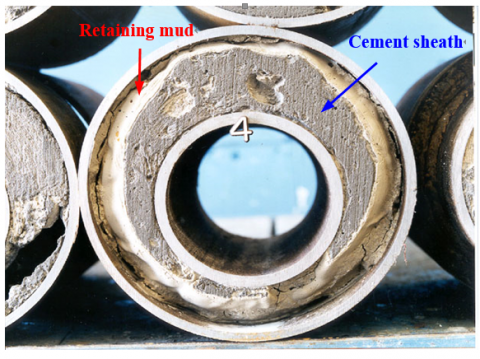Figure1. Local retention of drilling fluid on annular walls

3. Research on the Model of Drilling Fluid Non-retention in Eccentric Annulus

3.1 Research on the model for calculating boundary position of drilling fluid retention layer

It is well known that the flow when slurry displacing drilling fluid is complex. In the displacing process, the flow is influenced by not only fluids’ own non-Newtonian features, but also the physical, chemical and mechanical effects generated by fluids’ mixtures on the interface. Therefore, the flow process is very complex. In order to simply the problem, we assume that:

(1) Slurry contacts drilling fluid directly.

(2) Drilling fluid and slurry belong to Herschel-Bulkily fluids. The flow of slurry displacing drilling fluid satisfies laminar flow condition. And there exist non-slipping on the casing and well walls.

(3) The effects of fluids’ mixtures, diffusion and chemical reaction on the displacing flow are neglected.

(4) The effects of mud cake on the drilling fluid’s performances on the walls aren’t taken into consideration.

In cementing operation, the displacement interface will extend from the annular center to casing and well walls with time. When the driving force can’t overcome the yield stress of drilling fluid, the displacement will stop extending and at the time a steady displacement interface is generated. As shown in Fig.2, R1 is the annular inner diameter; R2 is the annular external diameter; r0 is the inner diameter of the flow core; R0 is the external diameter of the flow core; r1 and r3 represents the positions of drilling fluid retention layer boundary respectively, i.e. the interface between slurry and drilling fluid at any displacing time; r2 represents any position of the drilling fluid close to well wall, and r4 represents any position of the drilling fluid close to casing wall; q is the angle of the chosen displacing section.

## 2.png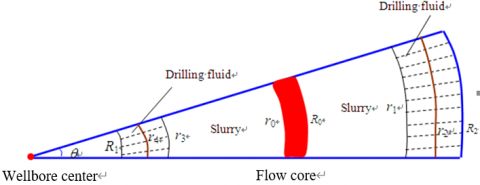Figure 2. The displacing section of annular slurry

A drilling fluid element, which is close to the well wall and its section angle is Dθ, is chosen as an analysis object. Although the drilling fluid element isn’t displaced by slurry, it still experiences the effects of the driving pressure, shearing stress, the driving force generated by density difference and its own yield stress. Furthermore, the driving pressure, shearing stress and driving force can facilitate the flow of drilling fluid, but the yield stress is resistance. In this paper, an element of drilling fluid whose height is DL is chosen for mechanical analysis between r1 and r2. The stress diagram is shown as Fig.3.

## 3.png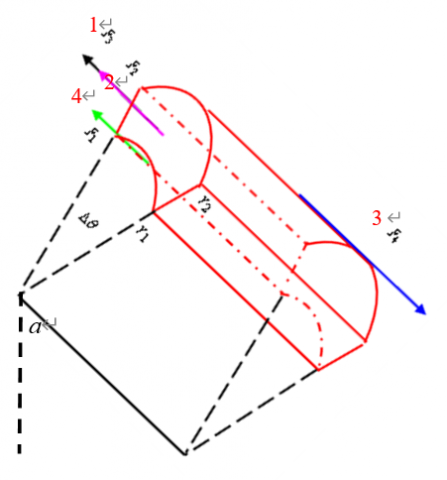Figure 3. Stress diagram of drilling fluid element

Refer to the literatures [15-16] and we can conclude that:

(1) The shearing stress born by drilling fluid is increasing with the increase of r2. It is easier to find that the shearing stress is the maximum on the well wall. So, the drilling fluid there is the easiest to be displaced. However, because displacing fluid satisfies stratified flow, which is different from the solid body, the displacement interface extends to the well wall stratifiedly and gradually. And r1 where the interface locates in will become bigger with the extension, until reach to the position R2, i.e. the well wall. In this situation, drilling fluid non-retention on the well wall can be achieved.

(2) On the other hand, the increase of r1 will result in the decrease of the shearing stress born by drilling fluid on the well wall. Therefore, we can summarize the mechanism of slurry displacing drilling fluid is that: In cementing operation, the shearing stress on the well wall is maximum, and after considering the stratified flow, the drilling fluid on the well wall begins flowing only when the displacement interface extends. Furthermore, before the flowing, the displacement interface will extend from the annular center to well wall stratifiedly. And the shearing stress born by the drilling fluid on the well wall is decreasing gradually with the interface extension. Until the shearing stress decrease to the yield stress, the displacement interface stops extending and a steady interface is generated. Finally, the drilling fluid between the interface and the well wall is retained.

Based on the force equilibrium condition and referred to [15-16], the boundary position of drilling fluid retention close to well wall can be expressed by:

$\Delta \rho g \sin \alpha \cos \theta r_{1}^{3}-\left[\Delta \rho g \sin \alpha(1+\cos \theta) R_{2}-\Delta \rho g \cos \alpha\right] r_{1}^{2}+ \Delta \rho g \sin \alpha R_{2}^{2} r_{1}+\Delta \rho g \cos \alpha R_{2}^{2}+\frac{1}{2} \frac{\Delta P}{\Delta L}\left(R_{2}^{2}-R_{0}^{2}\right)+\tau_{\mathrm{HBC}} R_{0}-\tau_{\mathrm{HBm}} R_{2}=0$   (1)

Similarly, the boundary position of drilling fluid retention close to casing wall can be expressed by:

$\Delta \rho g \cos \alpha r_{3}^{2}+\Delta \rho g R_{1} \sin \alpha\left(R_{2}-R_{1} \cos \theta\right) r_{3}+\tau_{\mathrm{HBC}} r_{0}-\tau_{\mathrm{HBm}} R_{1}+\frac{1}{2} \frac{\Delta P}{\Delta L}\left(r_{0}^{2}-R_{1}^{2}\right)+\Delta \rho g R_{1}^{2}\left[\sin \alpha \cos \theta R_{1}-R_{2} \sin \alpha-\cos \alpha\right]=0$ (2)

Where,

$R_{1}=-\varepsilon\left(R_{2}-R\right) \cos \theta+\sqrt{\varepsilon^{2}\left(R_{2}-R\right)^{2}\left(\cos ^{2} \theta-1\right)+R^{2}}$

Where, a is the deviation angle, °; Dr is the density difference between slurry and drilling fluid, g/cm3; R is the external diameter of casing, cm; e is the casing eccentricity, non-dimensional; q is the circumferential angle in the coordinate system which regards the annular wide gap as the origin, °; tHBc is the yield stress of slurry, Pa; tHBm is the yield stress of drilling fluid, Pa; DP/DL is the driving pressure difference, Pa/cm.

Furthermore, when a is zero, equations (1) and (2) are especially for the vertical well, and for the horizontal well when a =90º.

The thickness of drilling fluid retention on the well wall in different circumferential angles:

$h_{1}=R_{2}-r_{1} h_{1} \geq 0$    (3)

The thickness of drilling fluid retention on the casing wall in different circumferential angles:

$h_{2}=r_{3}-R_{1}=r_{3}+\varepsilon\left(R_{2}-R\right) \cos \theta-\sqrt{\varepsilon^{2}\left(R_{2}-R\right)^{2}\left(\cos ^{2} \theta-1\right)+R^{2}}$

$h_{2} \geq 0$     (4)

3.2 Analysis on the drilling fluid retention rules on casing and well walls

When the basic data, including τHBc=4 Pa, ΔPL=10Pa/cm, ρc=1.9g/cm3, ρm=1.3 g/cm3, R=6.985cm and R2=11.5cm, is given, the retention rules of drilling fluid in different circumferential angles can be described as shown in  Fig.4~7.

## 4.png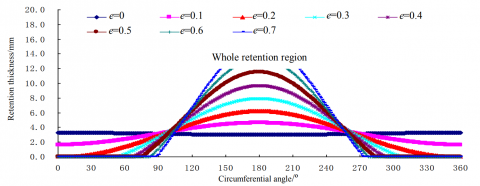Figure 4. The retention thickness on well wall in the vertical well

## 5.png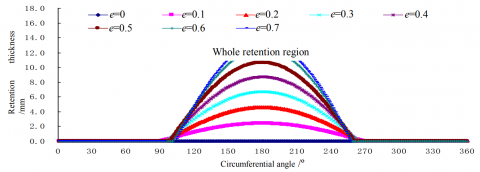Figure 5.The retention thickness on casing wall in the vertical well

## 6.png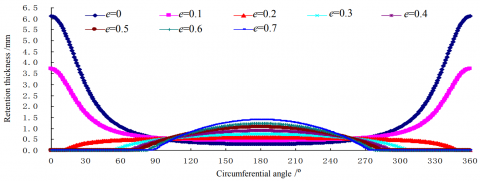Figure 6. The retention thickness on well wall at deviation angle 75

## 7.png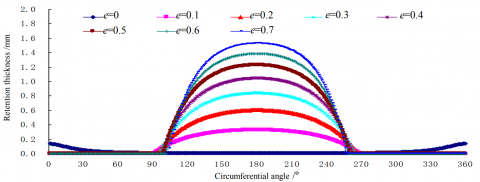Figure 7. The retention thickness on casing wall at deviation angle 75

From Fig.4~7, we can conclude that:

(1) For the vertical well, the maximum retention thickness of drilling fluid on casing and well walls is in the annular narrow gap. And the retention thickness on the well wall is bigger than that on the casing wall, or to say that the sealing effect on the cement-formation interface is weaker. When the casing eccentricity is increasing, the retention thickness on wide-gap walls decreases, but on the narrow-gap walls, it increases and the retention region decreases. So, increasing the casing centralizer can enhance the displacement effect and annular sealing quality in the vertical well.

(2) For the inclined well, the maximum retention thickness is increasing firstly and then decreasing with the increase of casing eccentricity. When casing eccentricity is smaller, the maximum retention is increasing with the increase of the inclined angle. But when the casing eccentricity reaches a certain point, the thickness is decreasing with the increase of the inclined angle.

(3) When the casing eccentricity is smaller in the inclined well, the position of the maximum retention thickness is on the up well wall of annular high side. And the position will transfer to the casing wall on annular low side with the increase of eccentricity. In generally, the retention region on the well wall is bigger than that on casing wall.

3.3 The condition model of drilling fluid non-retention on casing and well walls

The non-retention of drilling fluid means the displacing interface reaching the casing and well walls. So the non-retention condition on the well wall is:

$r_{1} \geq R_{2}$     (5)

When substituting equation (5) into (1), the condition model of drilling fluid non-retention on the well wall can be achieved:

$\tau_{H B m} \leq \tau_{\mathrm{IIBC}} R_{\mathrm{O}}+\frac{1}{2} \frac{\Delta P}{\Delta L}\left[R_{2}^{2}-\left(\frac{\sqrt{2\left(\frac{\Delta P}{\Delta L}\right)^{2}\left(R_{1}^{2}+R_{2}^{2}\right)}-4 \frac{\Delta P}{\Delta L} \tau_{\mathrm{HBC}}\left(R_{1}+R_{2}\right)+4 \tau_{\mathrm{HBC}}}{2 \frac{\Delta P}{\Delta L}}\right)^{2}\right] /R^{2}$    (6)

Similarly, the non-retention condition on the casing wall is:

r_{3} \leq R_{1}      (7)

When substituting equation (7) into (2), the condition model for drilling fluid non-retention on casing wall can be achieved:

$\tau_{H B m} \leq \tau_{\mathrm{IIBC}} R_{\mathrm{O}}+\frac{1}{2} \frac{\Delta P}{\Delta L}\left[R_{2}^{2}-\left(\frac{\sqrt{2\left(\frac{\Delta P}{\Delta L}\right)^{2}\left(R_{1}^{2}+R_{2}^{2}\right)}-4 \frac{\Delta P}{\Delta L} \tau_{\mathrm{HBC}}\left(R_{1}+R_{2}\right)}{2 \frac{\Delta P}{\Delta L}}\right)^{2}\right] -R_{1}^{2}/R^{2}$   (8)

As shown in equations (6) and (8), the critical condition of displacing interface reaching the walls is that the yield stress of drilling fluid is equal to the shearing stress when the annular space is full of slurry. At this situation, there exists no driving force generated by density difference. Therefore, we can conclude that the condition model of drilling fluid non-retention has nothing to do with the density difference, i.e. density difference can’t affect the range of drilling fluid retention. However, once the retention of drilling fluid happens, the retention thickness can be affected by the density difference. When the wellbore conditions is given, the range of drilling fluid retention can be decreased by increasing the driving pressure, the yield stress of slurry and reducing the casing eccentricity, the yield stress of drilling fluid. So, adjusting the parameters of fluids’ performances and cementing construction can enhance the displacing effect and cementing quality to some extent.

As shown in equations (6) and (8), the condition model of drilling fluid non-retention is a function about the inner and external diameter of slurry flow core. Therefore, it is necessary to obtain the expressions of the inner and external diameters.

There exists no velocity gradient in the flow core. Based on mechanism analysis on the element of flow core, the relationship between the inner and external diameters can be expressed by:

$R_{0}-r_{0}=\frac{2 \tau_{\mathrm{HBC}}}{\frac{\Delta P}{\Delta L}}$    (9)

If the flow of slurry is uniform in the axial direction, based on the kinematic equation of Herschel-Bulkely fluid and the same velocity in the inner and external diameters of flow core, another relationship between the two diameters is:

$\left[\frac{\Delta P}{\Delta L} r_{0}-\tau_{\mathrm{HBc}}\right]^{\frac{n+1}{n}}-\left[\frac{\Delta P}{\Delta L} R_{1}-\tau_{\mathrm{HBc}}\right]^{\frac{n+1}{n}}=\left[\frac{\Delta P}{\Delta L} R_{2}-\tau_{\mathrm{HBc}}\right]^{\frac{n+1}{n}}-\left[\frac{\Delta P}{\Delta L} R_{0}-\tau_{\mathrm{HBc}}\right]^{\frac{n+1}{n}}$     (10)

Where, n is the liquidity index of slurry.

When the driving pressure, inner and external diameters of annulus and slurry’s liquidity index are given, the inner and external diameters of flow core can be obtained by combining equation (9) with (10). Then substitute the calculation results into equation (6) and (8), the critical condition of drilling fluid non-retention in different circumferential angles can be achieved at last. However, because the calculating process is very complex, it isn’t suitable for field application. Another new method is necessary to explore.

Based on the model for calculating displacement interface boundary positions (r1, r3), r1 and r3 are related to the inner and outer radius (r0, R0). We know that the expressions of r0 and R0 are fractional exponent functions about liquidity index n. An analytical expression of r0 and R0 about n can’t be easily deduced. Fortunately, based on the equations’ features, we find that the value of n has little effect on r0 and R0 when satisfying R1/R2>0.3 . In order to see the effect degree of n, for the given casing eccentricity 0, 0.2, 0.4 and 0.6, we calculate r0 and R0 in annular wide and narrow gaps respectively when the value n is from 0.5 to 1.0. As a result, among the range of n values, the biggest differences of calculating r0 and R0 at the same casing eccentricity are less than 6 percent. We can conclude that the the value n really has little effect on r0 and R0. To simplify the calculation process, we choose the value of n as 1. So, the equation (10) can be expressed a binary quadratic equation about r0 and R0. By combining with equation (9), the analytical expressions of r0 and R0 are:

$r_{0}=\frac{\sqrt{2\left(\frac{\Delta P}{\Delta L}\right)^{2}\left(R_{1}^{2}+R_{2}^{2}\right)-4 \frac{\Delta P}{\Delta L} \tau_{\mathrm{HBc}}\left(R_{1}+R_{2}\right)}}{2 \frac{\Delta P}{\Delta L}}$      (11)

$R_{0}=\frac{\sqrt{2\left(\frac{\Delta P}{\Delta L}\right)^{2}\left(R_{1}^{2}+R_{2}^{2}\right)-4 \frac{\Delta P}{\Delta L} \tau_{\mathrm{HBc}}\left(R_{1}+R_{2}\right)}+4 \tau_{\mathrm{HBc}}}{2 \frac{\Delta P}{\Delta L}}$  (12)

When respectively substituting equations (11) and (12) into equations (6) and (8), the analytical expressions of drilling fluid non-retention condition on casing and well walls can be achieved. There is no doubt that the simplification is convenient to be used in field

4. Analysis on the Change Rules of Drilling Fluid Non-retention in Eccentric Annulus

The critical yield stress of drilling fluid non-retention in different circumferential angles can be calculated using the above models. As shown in Fig.8, the imaginary line represents the yield stress of chosen drilling fluid.

## 8a.png(a) eccentricity 0

## 8b.png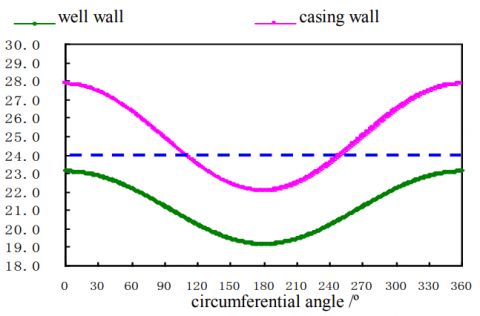(b) eccentricity 0.1

## 8c.png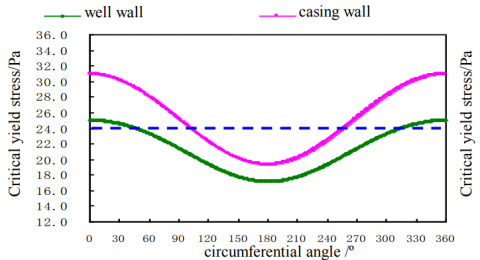(c) eccentricity 0.2

## 8d.png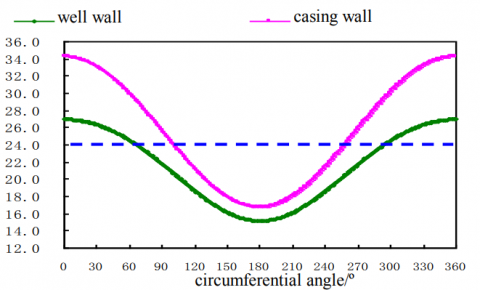(d) eccentricity 0.3

## 8e.png(e) eccentricity 0.4

## 8f.png(f) eccentricity 0.4

Figure 8. The critical yield stress of drilling fluid non-retention on casing and well walls

As shown in Fig.8:

(1) When the casing eccentricity is smaller, the range of drilling fluid retention on the well wall far exceeds that on the casing wall. But the range gap becomes smaller gradually with the eccentricity.

(2) The critical yield stress of drilling fluid non-retention in annular wide gap is increasing with the increase of casing eccentricity. However, for the narrow gap, the critical yield stress is decreasing. Therefore, it is easier for the wide gap to achieve non-retention displacement under the resistance effect caused by casing eccentricity. The displacing effect in the narrow gap will become worse.

(3) The total range of drilling fluid retention on casing and well walls is decreased as the eccentricity increased. Especially, for the annular narrow gap, the requested critical yield stress becomes smaller, and the retention degree will be more severe.

(4) The critical yield stress difference between the casing and well walls in the annular wide gap, will be increased as the eccentricity increased. But in the annular narrow gap, it will be decreased gradually.

5. Conclusions

(1) For the vertical well, the thickness of drilling fluid retention on the well wall is bigger than that on the casing wall, or to say that the sealing effect on the cement-formation interface is weaker. So, increasing the casing centralizer can enhance the displacement effect and annular sealing quality in the vertical well. For the inclined well, the position of the maximum retention thickness will transfer from the well wall on annular high side to the casing wall on annular low side with the increase of eccentricity.

(2) In this paper, an useful mathematical model for calculating drilling fluid non-retention on casing and well walls in eccentric annulus is established. Based on the model, two methods to enhance the cementing effects are provided: on the one hand, adjusting fluids’ performances and construction parameters on the basis of casing eccentricity, and on the other hand, depend on the non-retention condition of drilling fluid in annular narrow gap as the design basis.

(3) Density difference can’t affect the range of drilling fluid retention. However, once the retention of drilling fluid happens, the retention thickness can be affected by the density difference. When the wellbore conditions is given, the retention range of drilling fluid can be decreased by increasing the driving pressure, the yield stress of slurry and reducing the casing eccentricity, the yield stress of drilling fluid.

(4) The total range of drilling fluid retention on casing and well walls is decreased as the eccentricity increased. Especially, for the annular narrow gap, the requested critical yield stress becomes smaller, and the retention degree will be more severe.

(5) The critical yield stress differences between the casing and well walls in the  annular wide gap, will be increased as the eccentricity increased. But in the annular narrow gap, it will be decreased gradually.

Acknowledgements

The paper is supported by National Natural Science Foundation of China (Grant No. 51404071).

References

1. Azim Kalantariasl, Abbas Zeinijahromi, Pavel Bedrikovetsky et al. External filter cake buildup in dynamic filtration: mechanisms and key factors[C]. Lafayette, Louisiana, USA, 26-28 February 2014, SPE 168144.

2. M.A. Al-Ctaibi, K.H.BinMoqbil, A.S.Al-Rabba et al. Single-stage chemical treatment for oil-based mudcake cleanup: field case and labortory studies[C]. Lafayette, Louisiana, USA, 10-12 February 2010, SPE 127795.

3. L.A.Calcada, C.M.Schid, N.D.Calabrez, et al. A simplified methodology for dynamic drilling fluid filtration estimation considering mudcake compressibility[C]. Lafayette, Louisiana, USA, 26-28 February 2014, SPE 168208.

4. Reddy, Machireddy Gnaneswara. Influence of thermal radiation on natural convection boundary layer flow of a nanofluid past a vertical plate with uniform heat flux[J]. International Journal of Heat and Technology, 2014, 32(1): 1-7.

5. Pitakarnnop, Jeerasak. Slip flow in triangular and trapezoidal microchannels[J]. International Journal of Heat and Technology, 2009, 26(1): 167-174.

6. Chen Jialang, Liu yongjian. The ultimate allowable width of eccentric annular narrow gap in cementing[J]. Journal of Daqing Petroleum Institute, 1987, 11(4): 17-22.

7. Deng Jianmin. A calculation method on critical gel strength fluids for cementing without drilling fluid retention[J]. Natural Gas Industry, 2008, 28(5): 72-73.

8. Deng Jianmin, Wang Yonghong, Jia Xiaobin, etc. The method to calculate cementing fluids’ density and rheological parameter[J]. Petroleum Drilling Techniques, 2011, 39(5): 45-48.

9. Malekmohammadi S.; Carrasco-Teja M.; Storey S., Frigaard I.A.; Artinez D.M. An experimental study of laminar displacement flows in narrow vertical eccentric annuli[J]. J. Fluid Mech., 2010, 649, 371-398.

10. Frigaard I.A.; Pelipenko S. Effect of density difference on displacement efficiency during low-rate cementing[J]. Petroleum Drilling Techniques, 2007, 35, 79-82.

11. Pelipenko S.; Frigaard I.A. Visco-plastic fluid displacements in near vertical narrow eccentric annuli: prediction of travelling-wave solutions and interfacial in-stability[J]. Journal of Fluid Mechanics, 2004, 1-35.

12. Mukhopadhyay, Swati. Slip effects on mixed convection flow along a stretching cylinder[J]. International Journal of Heat and Technology, 2012, 30(2): 19-24.

13. Li Zhongmin, Wang Ruihe, Wang Chengwen, ect. A study on drilling mud stagnant layer thickness under the condition of laminar displacement[J]. Chinese Journal of Hydrodynamics, 2013, 28(5): 531-537.

14. Wang Ruihe, Li Zhongmin, Wang Chengwen, ect. Research progress in the cementing displacement mechanism[J]. Natural gas Industry, 2013, 33(5): 69-76. 15

15. Feng Fuping, Ai Chi, Yang Fengyu, ect. A study on the replacement position of laminar flow in eccentric annulus at retention layer boundaries[J]. Acta Petrolei Sinica, 2010, 31(5): 859-862.

16. Feng Fuping, Ai Chi, Peng Wanyong, ect. Effect of casing eccentricity on displacement in horizontal well[J]. Oil Drilling & Production Technology, 2011, 33(3): 12-16.

17. Wang Haige, Liu Xisheng, Ding Gang. Laminar flow of non-Newtonian fluids through eccentric annuli in horizontal section of wells[J]. Journal of the University of Petroleum China, 1993, 17(5): 26-34.